﻿ Multi-objective Optimization of Passive Shock Absorber for Landing GearPublications are Open
Access in this journal
Article Versions
Export Article
• Normal Style
• MLA Style
• APA Style
• Chicago Style
Research Article
Open Access Peer-reviewed

### Multi-objective Optimization of Passive Shock Absorber for Landing Gear

Fenghui Shi
American Journal of Mechanical Engineering. 2019, 7(2), 79-86. DOI: 10.12691/ajme-7-2-4
Received March 16, 2019; Revised April 27, 2019; Accepted May 03, 2019

### Abstract

In order to solve the mass variation problem of aircraft landing gears, usually, semi-active or active shock absorbers are considered first. In this study, however, several types of metering pin constructions are proposed, and have been obtained optimal solutions through multi-objective optimization. The multi-objective optimization methods to find optimum solutions of metering pin dimensions, displacements and the initial pressure of gas are proposed to deal with the mass variation problem. The optimum pareto front sets of four passive shock absorbers are compared and evaluated in this paper. Using the optimum solutions selected from the optimum pareto front sets, we also evaluate and compare the performance of each passive shock absorber with different metering pin construction through simulations. It is evident that the multi-objective optimization solutions of passive shock absorber with new metering pin constructions reduced the mass variation problem to a certain extent. The optimization results and method are helpful in the design of landing gears shock absorbers for various aircrafts.

### 1. Introduction

Oleo-pneumatic shock absorbers, namely passive shock absorbers are commonly used in medium/large aircraft landing gears, and can be optimally designed for specific conditions. However, passive shock absorbers cannot function optimally under conditions that differ from their specified conditions. This is called the mass variation problem of passive shock absorbers. Instead of passive shock absorbers, the active and the semi-active shock absorbers in landing gears are used providing good performance for both landing impact and taxi situations, while having the ability to adapt to various ground and operational conditions.

In Reference 1, Krüger focuses on optimization of taxiing performance of a semi-active landing gear. Ghiringhelli studies sensitivity of the complete aircraft model to the variation of control parameters and compares the results obtained in the simulated drop tests between passive and semi-active approach 2. Maemori 3 proposes an optimization method for a semi-active landing gear to handle variations in the maximum vertical acceleration of an aircraft during landing caused by the variation of the aircraft mass, which is always due to the variations in the number of passengers, and the amounts of cargo and fuel. In References 4, McGehee and Howell studied on the active landing gear was experimentally compared to a passive landing gear. In References 5, the semi-active landing gear was designed using multi-objective optimization which has been applied in various studies 6. In Reference 7, the possible optimization strategies for semi-active landing gear at touchdown and during taxiing were discussed. In addition, problems concerning mass variation have been discussed (Kobayashi, et al., 2009 8; Kobayashi, et al., 2013 9; Maemori, et al., 2003 3; Maemori, et al., 2004 10 and SHI, et al., 2016 11) and in References 11, a bypass shock absorber was discussed and tested to deal with the mass variation problem.

Active control and semi-active control are widely used approach in many field of vibration control. However, compared with passive control, active and semi-active control have excellent tunable ability due to their flexible structure. Active control needs an external hydraulic source to supply energy for the system, have complex constructions and require a complex control system. They also have high costs, and breaking risks. It is especially difficult to install active or semi-active shock absorbers on smaller aircrafts.

On the other hand, oleo pneumatic passive shock absorbers are highly efficient as they can absorb and remove vertical kinetic energy simultaneously. This is due to the combination of the pneumatic spring force from the compression of the gas, and the damping or removal of energy due to the flow of hydraulic fluid through an orifice element. This combination of elements allows the system to be compact and highly efficient. The main resistance during landing is the dynamic resisting force depending on the orifice area. Although the orifice is merely a hole in the orifice plate, most designs have a metering pin extending through it, and by varying the pin diameter, the orifice area will also vary. This variation is adjusted so that the strut load is constant under dynamic loading.

In this paper, in order to reduce the mass variation problem to make the passive shock absorber have the same performance as the active or semi-active shock absorbers to a certain extent. Several types of metering pin constructions and some diameter approximate equation are proposed, especially multi-tapered, single-parabolic and multi-parabolic profiles metering pin are considered applying in the shock absorber of landing gear is the first time. Multi-objective optimization method, which to define the maximum vertical acceleration of aircraft at maximum and minimum masses as the multi-objective function is proposed in this research.

### 2. Shock Absorber

In this study, we deal with metering pins type shock absorber (Figure 1). The shock absorber that consist of steel cylinder 1 and rod 2 which move in the cylinder in two guide bearings 3 and 4. Guide bearing 3 is rigidly attached to the rod and moves together with it, and guide bearing 4 is attached to the cylinder. Between the inner surface of the cylinder 1 and the outer surface of the rod 2, there is a sealing ring 5. The guide bearing attached to the rod has holes through which the damping fluid can flow into the space between the cylinder and the rod. Inside the cylinder, there is tube plunger 6 with holes for the damping fluid to flow through. The plunger is attached to the top lid of the cylinder. Between the internal surface of the cylinder and rod, back valves 7 are placed to hamper the flow of the liquid into this space during the backward movement of the rod, thus increasing the damping effect.

The damping fluid partially fills the system as shown in Figure 1. Above the liquid, at the top part of the cylinder, there is a gas, which is compressed by the liquid when the rod moves into the cylinder and elastically resists this motion. Hence, this shock absorber combines a hydraulic damper and a pneumatic spring into one unit. The column of the fluid moving in the cylinder together with the rod plays the role of the piston of the pneumatic spring.

When the external force is applied to the shock absorber, for example, by the wheels of the landing gear, the rod moves into the cylinder, and the damping fluid partially fills the chamber, compressing the gas. This leads to the elastic reaction of the pneumatic spring. As the rod moves, the fluid flows through the holes in the tube plunger producing the damping effect. A metering pin with a variable cross section is attached to the rod (Figure 1). As the rod moves, the metering pin moves through the hole and changes the clearance through which the fluid can flow. By appropriately profiling the metering pin diameters along its length, we can provide the required relationship for orifice areas. During light loadings, such as landing at minimum mass, the orifice cross section is large, thus reducing the forces during these perturbations. During high loading the orifice cross section reduces accordingly and the dampening force increases.

In this study, three new construction of metering pin are proposed (Figure 2 (b), (c) and (d)).

• Figure 1. Schematic diagrams of passive shock absorber with metering pin
• Figure 2. Schematic diagrams of the metering pin constructions

### 3. Aircraft Landing Gear Model

3.1. Aircraft Weights

We deal with the Japanese national line Boeing 747-400 as the model. According to the JAL Practical Dictionary Flight Plan, Aircraft Specifications and Ability Table 11, the maximum design landing weight (MDLW) is 260.4t. The MDLW is the maximum certificated design weight for landing limited by aircraft strength and airworthiness requirements. It generally depends on the landing gear strength or the landing impact loads on certain parts of the wing structure. The manufacturer’s empty weight (MEW) is 164.3t, so the minimum landing weight can be expressed as 164.3t + 5t (reserve fuel).

Hence, in this paper, the maximum mass is defined as m1max=130t and the minimum mass as m1min = 85t for one landing gear. The performance of masses m1max =130t and m1min = 85t are discussed through simulations.

3.2. Resisting Forces of Shock Absorbers and Equations of Motion

The pneumatic spring resisting force fK of the shock absorber is expressed as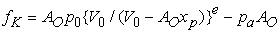(1)

where p0 and V0 are the initial pressure and volume of the gas in upper chamber respectively, and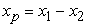(as shown in Figure 3).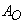is the effective pressurized area for outer diameters of the shock absorber. e is the polytropic index of gas inside the shock absorber. pa is the atmospheric pressure.

• Figure 3. Model of an aircraft landing gear

The resisting force fD due to the dynamic pressure is expressed as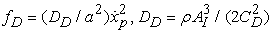(2)

where a is the orifice area (in Figure 1 it is expressed as axp).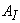is the effective pressurized area for inner diameters of shock absorber. CD is the discharge coefficient of orifice area, and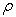is the density of fluid.

The total resisting force f of the shock absorber is expressed as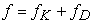(3)

When the lift acting on masses m1 and m2 is equal to the gravitational force acting on these masses, the equation of motion for the two-mass system shown in Figure 3, is expressed as

When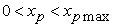, and as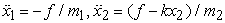(4)

When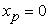or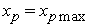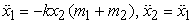(5)

Where m1 is the mass of aircraft including passengers, cargo, and fuel of one landing gear. m2 is the mass of tires and moving part of the shock absorber. k is the total spring constant of tires.

The orifice area (Figure 1) is calculated using Eq. (6).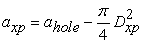(6)

Where, ahole is the hole area which the metering pin going through and axp is the orifice area which subtract metering pin cross-section area depends on displacement xp from the hole area. Dxp is the metering pin diameter depending on displacement xp, is calculated in the section 4.2

### 4. Optimization Problem

In this study, we do optimization about the passive shock absorbers with metering pin (Figure 1). The multi-objective function of optimization is to minimize the maximum vertical acceleration of the aircraft maximum mass m1,min and minimum mass m1,max. As the design variables, the diameters of the tapered (Figure 2 (a) and (b)), and parabolic (Figure 2 (c) and (d)) profiles metering pin, the initial pressure P0 (Eq. (1)) and the first step of displacement xp1, the second step of displacement xp2 (Figure 2 (b) and (d)) are optimized at masses 85t and 130t. We used MATLAB/Simulink 2015, and Global Optimization Toolbox to run simulations and optimizations in this work.

The parameters used in the calculations are, the effective pressurized area for inner and outer diameters of shock absorber piston tube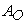= 0.123m2,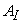=0.059 m2, the discharge coefficient of orifice area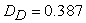kg･m3, the total spring constant of tires k = 4.3×106 kN/m, the mass of tires and moving part of shock absorber m2 = 1.8×103 kg, the polytropic index of gas inside shock absorber e = 1.1, the atmospheric pressure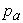= 1.01325×105 Pa, the aircraft landing speed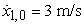, the maximum displacement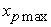= 0.65 m, and the area of the hole (Figure 1)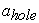= 0.0078 m2.

The Global Optimization toolbox from MATLAB 2015 are used in order to conduct the optimization. The gamultiobj solver creates four sets of Pareto front for a multi-objective minimization using the genetic algorithm.

4.1. Multi-objective Function

The multi-objective function is to minimize the maximum vertical acceleration of aircraft mass at m1=85t and m1=130t, expressed as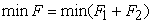(7)

where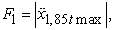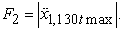The design variables are descripted and defined below in section 4.2

4.2. Design Variables

In this study, including diameters di of metering pin, I also proposed the displacements xp1, xp2 (Figure 2 (b) and (d)) and the initial pressure of gas inside shock absorber P0 (Eq. (1)) are the design variables. Because the displacements xp1, xp2 and P0 are relationship each other. If P0 becomes greater, the displacements xp1 and xp2 will become shorter in the same landing condition. The step displacements xp1, xp2 and the initial pressure of gas P0 must have the optimum values for the aircraft mass 85t and 130t.

4.2.1. Single-Taper Profile

In this section, the orifice area axp, which depends on displacement xp during landing when the mass is m1 =85t (minimum mass) and 130t (maximum mass), is optimized to find the optimal solutions for diameters d1, d2 (Figure 2(a)) and the initial pressure P0. The diameters calculation is expressed as Eq. (8).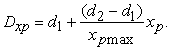(8)

4.2.2. Three-Taper Profile

The metering pin is divided into three-tapered profiles (Figure 2(b)). The bottom of the first taper is the top of the next tapered profile, connecting to each other. For the diameters di, the design variable numbers are i =1, 2, 3, 4. Design variables are diameters di and P0. The diameters of the three-tapered are expressed as Eq. (9), (10) and (11).

When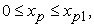the diameter is expressed as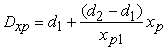(9)

When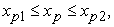the diameter is expressed as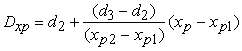(10)

When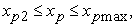the diameter is expressed as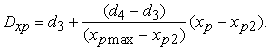(11)

4.2.3. Single-Parabolic Profile

In this section, we proposed a single-parabolic curved metering pin and diameter approximate equation. There are four design variables d1, d2, d3 and P0 in this optimization problem. The design variables d1, d2 and d3 are the top, middle and bottom diameters respectively (Figure 2(c)). The calculation for the diameter of the metering pin is expressed as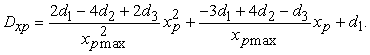(12)

4.2.4. Three-Parabolic Profile

In this section, I proposed a three-parabolic curved metering pin and diameter approximate equations. There are ten design variables d1, d2,… d7, xp1, xp2 and P0 in this optimization problem. Similar to section 4.2.2, the metering pin is divided into three parts, three-parabolic profiles (Figure 2(d)). The bottom of the first parabolic is the top of the next parabolic profile, connecting to each other. The diameter of the three parts parabolic expressed as Eq. (13), (14) and (15).

Whenthe diameter is expressed as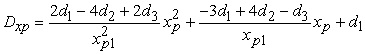(13)

Whenthe diameter is expressed as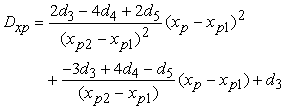(14)

Whenthe diameter is expressed as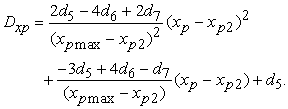(15)

### 5. Optimization Results

Figure 4 is comparison optimum pareto front sets of four kind shock absorber with different metering pin construction. It is clear that each pareto front sets is arranged ideally. The pareto front set of multi-taper is the smallest (red star), and single-taper is the biggest (red triangles).

• Figure 4. Comparison of Pareto fronts for multi-objective optimization solutions

For multi-taper (Figure 4 red stars sets) and multi-paraboric (Figure 4 blue stars sets), 70 multi-objective optimal results are obtained respectively. The middle of each solutions sets are the better multi-objective solutions, and be very close each other. On the ends of each pareto front sets, solutions are separated, same as single-objective optimal solutions optimized at mass 85t or 130t.

For single-taper (Figure 4 red triangles sets) and single-paraboric (Figure 4 blue reverse triangles sets), 18 optimal results are obtained respectively. The pareto sets fronts of single-taper and single-parabolic are separated clearly. The solutions of single-parabolic are better than single-taper.

For the multi-taper and the multi-parabolic, almost same optimal results obtained in the middle of the pareto front sets, and be found out little solutions function values than single-taper and single-parabolic. This is because the diameters of metering pin can change in detail along the displacement.

Figure 5 and Figure 6 show the details of pareto front sets of the single-taper and the single-parabolic metering pin constructions. 6 optimum solution are selected from 18 feasible solutions respectively, shown in Figure 5 and Figure 6. The optimum design variables, the diameters of metering pin and the initial pressure of gas P0 are shown in Table 1 and Table 2.

Figure 7 and Figure 8 show the details of pareto front sets of the multi-taper and the multi-parabolic metering pin. Six optimum solutions are selected from 70 feasible solutions respectively, shown in Figure 7 and Figure 8. The optimum design variables obtained, the diameters of metering pin, step displacements xp1, xp2 and the initial pressure of gas P0 are shown in Table 3 and Table 4.

The details of six optimal solutions of each metering pin are shown in Table 1, Table 2, Table 3 and Table 4. Approximate value of the initial pressure of gas P0 of single-taper and multi-taper are 6.8×105Pa and 6.6×105Pa.

• Figure 5. Pareto front of single-taper
• Figure 6. Pareto front of single-parabolic
• Figure 7. Pareto front of multi-parabolic
• Figure 8. Pareto front of multi-taper

Approximate value of the single-parabolic and the multi-parabolic are 7.7×105Pa and 8.4×105Pa, greater than both single-taper and three-taper type metering pins.

About the first and second step displacement xp1 and xp2, the second step displacement xp2 of multi-taper and multi-parabolic are almost same as approximate value 0.55m (Table 3 and Table 4), but the first step displacement xp1 are great different, xp1 of the multi-taper is average 0.13m, the multi-parabolic is average 0.35m (Table 3 and Table 4).

In Table 1, all solutions of the single-tapered metering pin, d2 is greater than d1, so the shapes are tapers along the displacement, but the tapering angles is very small. This means that the diameters do not need to be changed widely along the displacement. In Table 3, for the three-tapered metering pin, there are the taper or reverse taper shape for different step. This means that the orifice areas can change in detail than the single-tapered metering pin.

In order to confirm the performance of the optimum solutions, three solutions of each type of metering pin construction are selected from the pareto sets to simulate, and to be plotted in Figure 9 to Figure 12. They are the solutions, No.3, No.7 and No.12 of the single-taper (Figure 5 and Table 1), No.1, No.2 and No.4 of the single-parabolic (Figure 6 and Table 2), No.3, No.5 and No.64 of multi-taper (Figure 7 and Table 3), No.5, No.13 and No.29 of multi-parabolic (Figure 8 and Table 4).

As an explaining example of Figure 9, Figure 10, Figure 11 and Figure 12, Figure 12 is comparison of accelerations for three multi-parabolic solutions No.5, No.23 and No.29. The blue solid and broken lines are the solution No.5 simulating the accelerations for aircraft masses 130t and 85t (Figure 12), the results are conventional as same as single-objective optimization results at 130t, because that the blue solid line is ideal solution of 130t, almost constant from starting to the end of the line. The green solid and broken lines are the solution No.23 simulating the accelerations for aircraft masses 130t and 85t (Figure 12), have conventional solutions as same as single-objective optimum results at 85t, because that the green broken line (85t) is ideal solution of 85t, almost constant from starting to the end of the line.

• Figure 9. Comparison of single-taper solutions
• Figure 10. Comparison of single-parabolic solutions

The red solid and broken lines (Figure 12) are the simulation of the solution No. 29 (Figure 7), which is in the middle of the pareto front set between the solution No. 5 and No. 23 (Figure 7). About the red broken lines, the maxmum acceleration value is decreased 13.69% (see Figure 12) than blue broken line, be only 8.533% greater than green broken line (ideal solution of 85t), and almost parallel to the green broken line(ideal solution of 85t).

About the solid red lines, the maxmum acceleration value is decreased 11.45% than the green solid line, and only be 8.708% greater than blue solid line (ideal solution of 130t). The red solid line becomes bigger along the displacement smoothly, approach parallel to the blue solid line (ideal solution of 130t).

The optimum solutions of multi-objective optimization are not ideal results to deal with aircraft mass 130t and 85t like the results of single-objective optimization completely, but the performance of results are very good.

• Figure 11. Comparison of multi-taper solutions
• Figure 12. Comparison of multi-parabolic solutions
• Figure 13. Metering pin diagrams of optimal solutions examples

Figure 13 is the diagrams examples of metering pins with single-taper (solution No.12), three-taper (solution No.64), single-parabolic (solution No.4) and multi-parabolic (solution No.6) profiles optimal solutions. For the single-taper Figure 13 (a), the tapering angle is just 0.33850, the metering pin shape is close to a cylindrical column. For the three-tapered metering pin construction, the first step is a reverse taper, the second step is a taper, and the third is a reverse taper (Figure 13 (b)). This means that the orifice areas can vary in detail than the single-taper metering pin.

For the parabolic metering pin, if the middle diameter d2 is smaller or greater than top diameter d1 or bottom diameters d3 (Figure 2 (c)), the profiles will become concave or convex respectively. For example, Figure 13 (c) is concave curve. Figure 13 (d) is the optimized three-parabolic profiles, which have multi-convex and multi-concave geometry on the same metering pin. The first step is concave curve, the second step is half-convex and half-concave and the third step is convex. Although there are three parabolic profile parts, there are actually seven diameter design variables and three different convex or concave profiles that change the orifice areas finely and smoothly along the displacement.

### 6. Conclusions

In this study, in order to reduce the mass variation problem of passive shock absorbers, four type of metering pins in passive shock absorber including conventional taper pin, proposed the multi-taper, the single-parabolic and multi-parabolic metering pin constructions are considered. Each Eighteen optimal solutions for the single-tapered and the single-parabolic, each seventy optimal solutions for the multi-tapered and multi-parabolic metering pins are obtained through multi-objective optimization.

In comparison of optimum results of pareto optimal sets for four kinds of shock absorber with different metering pin construction, the multi-tapered and multi-parabolic metering pins have better solutions sets than the single-tapered and the single-parabolic.

It is not possible to obtain ideal results for every different impact condition, but the metering pin can be shaped based on one particular important landing case to improve the other landing conditions. For the single-tapered metering pin, there are tapered shape depending on the displacement. Nevertheless, whichever tapered, their tapering angles are very small. For single-parabolic metering pins, there are convex parabolic and concave parabolic shapes depending on the displacement. Regardless, the parabolic curve is very small. This means that the diameters do not need to be changed drastically along the displacement of the shock absorber.

For the three-taper and the three-parabolic profiles, based on the optimal solutions there are probably taper or reverse taper and the convex or the concavet parabolic for each step along the displacement. The diameters of the metering pins can be changed in detail than single-taper and single-parabolic along the displacement, while getting better solutions easier, having better performance and higher efficiency during the landing.

### References

  Krüger, W., Besselink, I., Cowling, D., Doan, D. B., Kortüm, W., and Krabacher, W., Aircraft Landing Gear Dynamics: Simulation and Control, Vehicle System Dynamics, 28, 1997, pp. 119-158. In article View Article  Ghiringhelli, L. G. (2000). Testing of semiactive landing gear control for a general aviation aircraft. Journal of Aircraft, Vol.7, No.4, pp. 606-616T. Eckes, The Developmental Social Psychology of Gender, Lawrence Erlbaum, 2000. [E-book] Available: netLibrary e-book. In article View Article  Maemori, K., Tanigawa, N., Koganei, R., and Morihara, T., 2003, Optimization of a Semi-Active Shock Absorber for Aircraft Landing Gear, Proc. DETC’03 ASME 2003 Design Engineering Technical Conferences and Computer and Information in Engineering Conference, Chicago, IL, DETC2003/DAC-48765. In article View Article  McGehee, J. R., and Dreher R. G., Experimental Investigation of Active Loads Control for Aircraft Landing Gear, NASA-TP-2042, 1982. In article  Krüger, W., Design and Simulation of Semi-Active Landing Gears for Transport Aircraft, Mechanics of Structures and Machines, 30(4), 2002, pp. 493-526. In article View Article  Altuzarra, O., Hernandez, A., Salgado, O., and Angeles, J., Multiobjective Optimum Design of a Symmetric Parallel Schönflies-Motion Generator, ASME J. Mech, Des., 131(3), 2009, pp. (1002, 1-11). In article View Article  Wang, X., and Carl, U., Fuzzy Control of Aircraft Semi-active Landing Gear System, AIAA-99-0265, 1999. In article View Article  Kobayashi, M., Shi, F., and Maemori, K., 2009, Modeling and Parameter Identification of a Shock Absorber Using Magnetorheological Fluid, J. System Design and Dynamics, 3(5), pp. 804-813. In article View Article  Kobayashi, M., Shi, F., and Maemori, K., Parameter Identification and Optimization of Shock Absorber Using Magnetorheological Fluid, Journal of System Design and Dynamics, 7(3) , 265-277, 2013. In article View Article  Maemori, K., Tanigawa, N., and Shi, F., Optimization of a Semi-Active Shock Absorber Using a Genetic Algorithm, Proc. DETC’04 ASME 2004 Design Engineering Technical Conferences and Computer and Information in Engineering Conference, Salt Lake City, Utah, DETC2004-57115, 2004. In article  Shi, F., Tanigawa, N., Koganei, R., and Maemori, K., Optimum Trade-Off Charts Considering Mass Variation for the Design of Semi-Active and Passive Shock Absorbers for Landing Gear, Journal of Advanced Mechanical Design, Systems, and Manufacturing, 10(1), 1-15, 2016. In article View Article  JAL Practical Dictionary: Flight plan, Aircraft specifications and Ability Table. http://www.jal.com/ja/jiten/dict/p435.html. In article  Howell, W. E., McGehee, J. R., Daugherty, R. H., and Vogler, W. A., F-106B Airplane Active Control Landing Gear Drop Test Performance, SAE Technical Paper Series, 901911, 1990. In article View ArticleThis work is licensed under a Creative Commons Attribution 4.0 International License. To view a copy of this license, visit http://creativecommons.org/licenses/by/4.0/